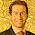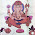## Monday, December 16, 2013

### PCA on NIFTY stocks

Principal component analysis can help to reduce a complex data set to a lower dimension to highlight a hidden structure. It is used to convert a set of observations of correlated variables to a set of values of uncorrelated variables called principal components. These principal components are linear combinations of the actual variables. The linear combinations are chosen in such a manner that the first principal component has the largest possible variance. The successive principal components have the largest amount of variance given that they are orthogonal to the preceding principal components. Hence the variance explained by the first few components tells us about the strength of the underlying trend. An implicit assumption here is that large variance represents important dynamics of the variables.

PCA on NIFTY stocks
NIFTY is one of the most widely tracked index for Indian equity market. It consists of 50 major Indian companies. PCA is used to analyze the correlated returns of these 50 stocks. The observations matrix consists of normalized daily returns of Nifty 50 stocks sampled from February to November 2013. Below is the Scree plot of the resulting principal components:

The first principal component is typically assumed to represent the broad market. The next few are assumed to be the sector/style related factors. The remaining components represent the idiosyncratic properties of stocks. For the given set of NIFTY stocks we can conclude that about 35% of all the variance is because of the broad market factor (systematic risk). Also about 15% of the variance can be explained by the sector/style related factors. The remaining 50% corresponds to the stock specific factors (unsystematic risk).  Below is the factor correlation plot:

While performing PCA, an important thing to look for is the variation of variance explained by the principal components over time.

Looking at the above plots, we can say that barring extreme rises in the broad market, stocks tend to fall together and rise independently of each other. This means that the component of market risks is higher on the downside. Also over the last couple of years, the sector/style factors have gotten stronger. It seems that the investing paradigm has shifted from timing markets to taking stock/sector level calls.

1.Good post. But how can I use this for trading? Also what is the correlation of these factors with Index?

2.How do you monitor the change of variance explained over time? is it just the square of the correlation on a rolling window?

1.Variance of the first principal component is given by the largest eigenvalue, so in order to find the dynamics of the variance of the PC1, one need to consider a rolling window first and apply PCA on it. The size of the rolling window can be decided with the help of kaiser's rule, see the book by jollife

3.How do you monitor the change of variance explained over time? is it just the square of the correlation on a rolling window?